# Recursion Examples Recursion review Recursion review Scenario You

• Slides: 29Recursion ExamplesRecursion (review)Recursion (review) Scenario: You are waiting in line for a concert. You can't see the front of the line, but you want to know your place in the line. The person at the front, knows they are at the front! Base case You ask the person in front of you: “what is your place in the line? ” Recursive call When the person in front of you figures it out and tells you, add one to that answer. Use the solution to the smaller problemIteration vs. Recursion ● Iteration and recursion are somewhat related ● Converting iteration to recursion is formulaic, but converting recursion to iteration can be more tricky Iterative Recursive def fact_iter(n): def fact(n): total, k = 1, 1 if n == 0: while k <= n: total, k = total*k, k+1 return total Names: n, total, k, fact_iter return 1 else: return n * fact(n-1) Names: n, factSum Digits Let’s implement a recursive function to sum all the digits of `n`. Assume `n` is positive. def sum_digits(n): """Calculates the sum of the digits `n`. >>> sum_digits(8) 8 >>> sum_digits(18) 9 >>> sum_digits(2018) 11 """ ***" "*** YOUR CODE HERE 1. One or more base cases 2. One or more recursive calls with simpler arguments. 3. Using the recursive call to solve our larger problem.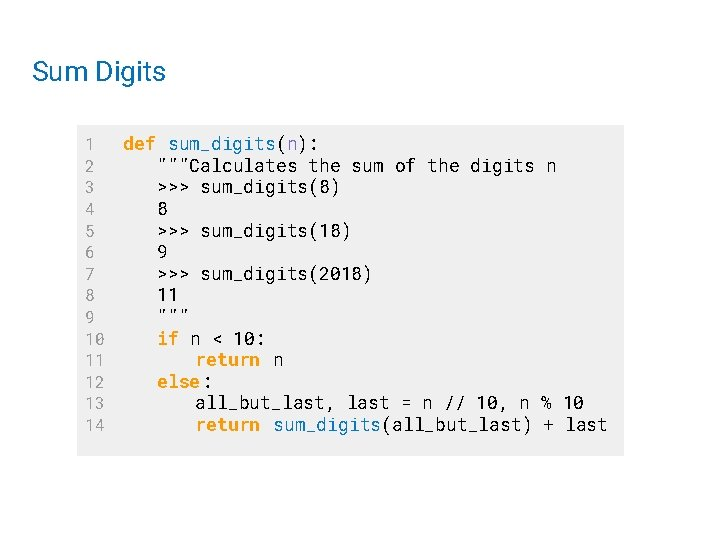Sum Digits 1 2 3 4 5 6 7 8 9 10 11 12 13 14 def sum_digits(n): """Calculates the sum of the digits n >>> sum_digits(8) 8 >>> sum_digits(18) 9 >>> sum_digits(2018) 11 """ if n < 10: return n else: all_but_last, last = n // 10, n % 10 return sum_digits(all_but_last) + lastOrder of Recursive CallsDemo Cascade Goal: Print out a cascading tree of a positive integer n. >>> cascade(486) 486 48 486 >>> cascade(48) 48 4 48 >>> cascade(4) 4 Ideas: ● If n is a single digit, just print it out! ● Otherwise, let cascade(n // 10) take care of the middle and print(n) around it 11 22 33 4 5 6 7 def cascade(n): if n < 10: print (n) else: print (n) cascade(n // 10) print (n)The Cascade Function Output 123 12 1 12 Base case Each cascade frame is from a different call to cascade. Until the Return value appears, that call has not completed. Any statement can appear before or after the recursive call.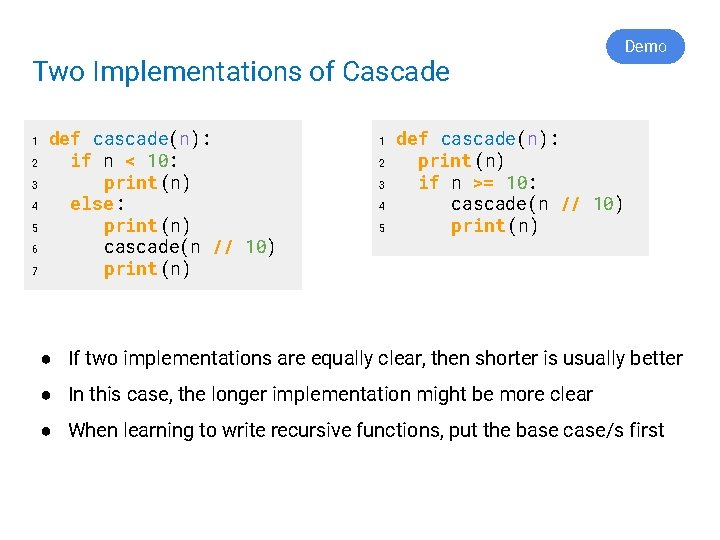Two Implementations of Cascade 1 2 3 4 5 6 7 def cascade(n): if n < 10: print (n) else: print (n) cascade(n // 10) print (n) 1 2 3 4 5 Demo def cascade(n): print (n) if n >= 10: cascade(n // 10) print (n) ● If two implementations are equally clear, then shorter is usually better ● In this case, the longer implementation might be more clear ● When learning to write recursive functions, put the base case/s firstFibonacci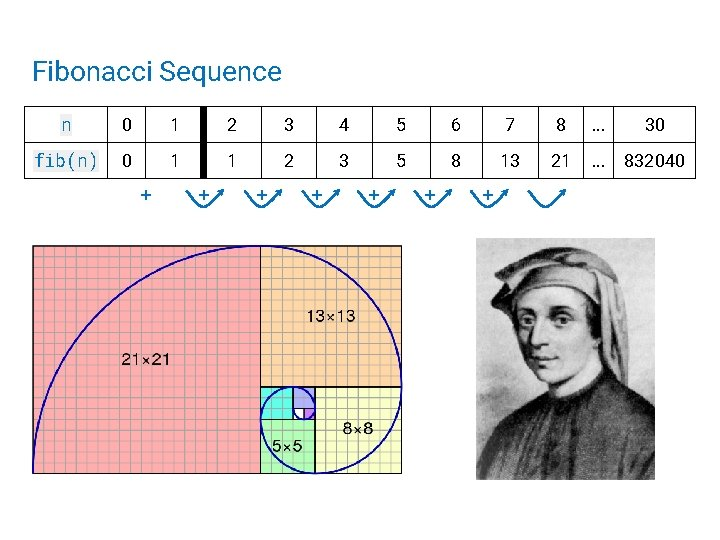Fibonacci Sequence n 0 1 2 3 4 5 6 7 8 . . . 30 fib(n) 0 1 1 2 3 5 8 13 21 . . . 832040 + + + +fib(1) == 1 fib(2) == 1 fib(3) == 2 fib(4) == 3 fib(5) == 5 fib(6) == 8 Fibonacci's rabbits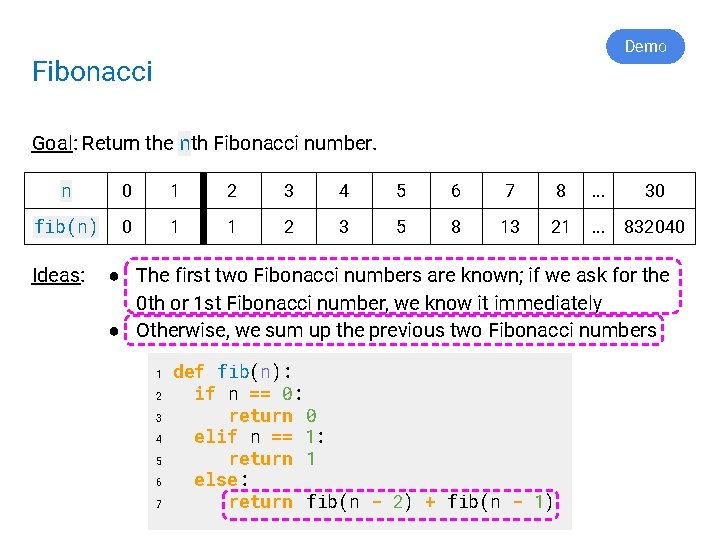Demo Fibonacci Goal: Return the nth Fibonacci number. n 0 1 2 3 4 5 6 7 8 . . . 30 fib(n) 0 1 1 2 3 5 8 13 21 . . . 832040 Ideas: ● The first two Fibonacci numbers are known; if we ask for the 0 th or 1 st Fibonacci number, we know it immediately ● Otherwise, we sum up the previous two Fibonacci numbers 1 2 3 4 5 6 7 def fib(n): if nif == n 0: == 0: return 0 elif n == n 1: == 1: return 1 else: return fib(n - 2) + fib(n - 1)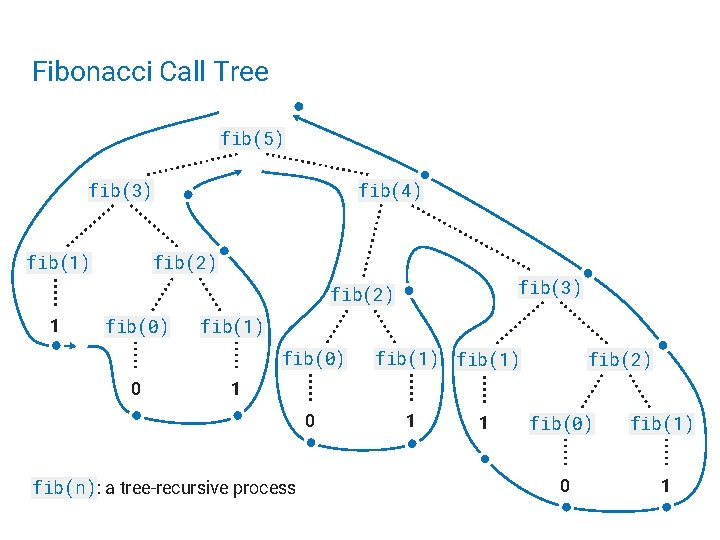Fibonacci Call Tree fib(5) fib(3) fib(1) fib(4) fib(2) fib(3) fib(2) 1 fib(0) fib(1) fib(0) 0 fib(1) fib(2) 1 0 fib(n): a tree-recursive process 1 1 fib(0) fib(1) 0 1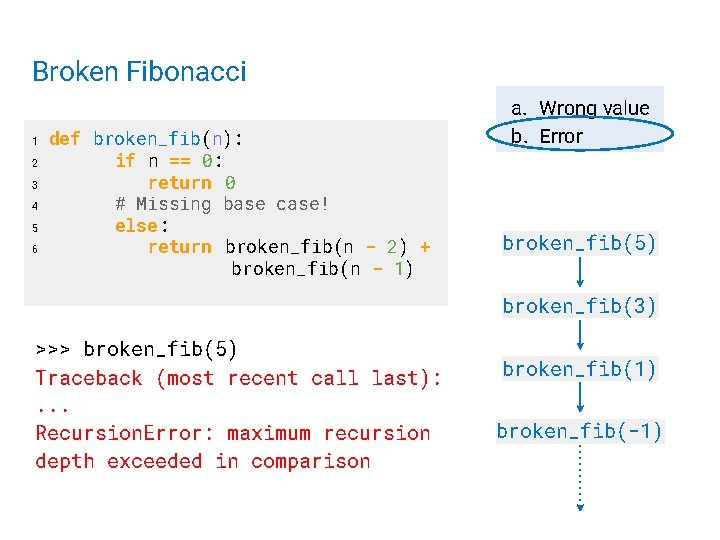Broken Fibonacci def fib(n): broken_fib(n): 2 if n == 0: return 0 3 return 0 elif n == base 1: 4 # Missing case! 5 else: return 1 else: 6 return broken_fib(n - 2) + 7 fib(n - 1)- +1) ………………. . return broken_fib(n - 2) 1 >>> broken_fib(5) Traceback (most recent call last): . . . Recursion. Error: maximum recursion depth exceeded in comparison a. Wrong value b. Error broken_fib(5) broken_fib(3) broken_fib(1) broken_fib(-1)fib(5 ) fib(3 ) fib(1 ) fib(-1) fib(-3) Broken fib(n) Never computed!Counting Partitions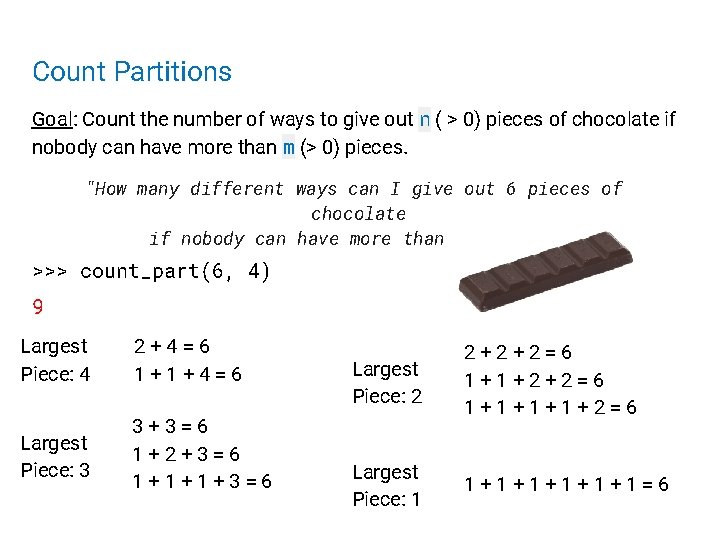Count Partitions Goal: Count the number of ways to give out n ( > 0) pieces of chocolate if nobody can have more than m (> 0) pieces. "How many different ways can I give out 6 pieces of chocolate if nobody can have more than 4 pieces? " >>> count_part(6, 4) 9 Largest Piece: 4 2+4=6 1+1+4=6 Largest Piece: 3 3+3=6 1+2+3=6 1+1+1+3=6 Largest Piece: 2 2+2+2=6 1+1+1+1+2=6 Largest Piece: 1 1+1+1+1=6Count Partitions 2+4 1+1+4 3+3 1+2+3 1+1+1+3 2+2+2 1+1+1+1+2 1+1+1+1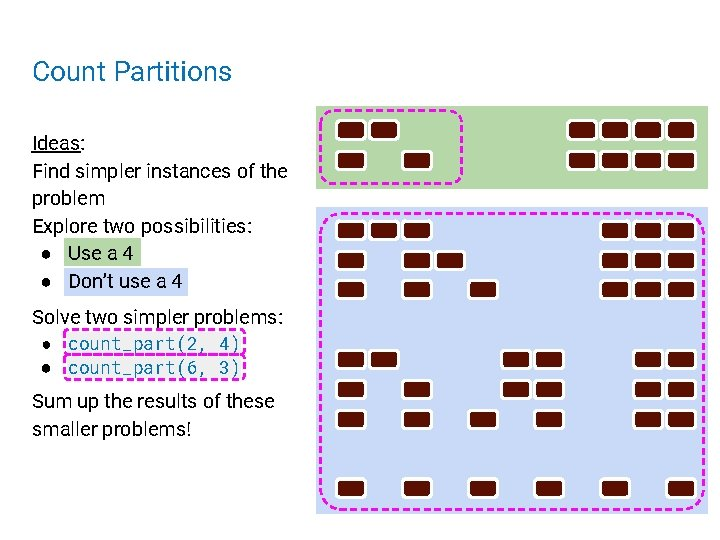Count Partitions Ideas: Find simpler instances of the problem Explore two possibilities: ● Use a 4 ● Don’t use a 4 Solve two simpler problems: ● count_part(2, 4) ● count_part(6, 3) Sum up the results of these smaller problems!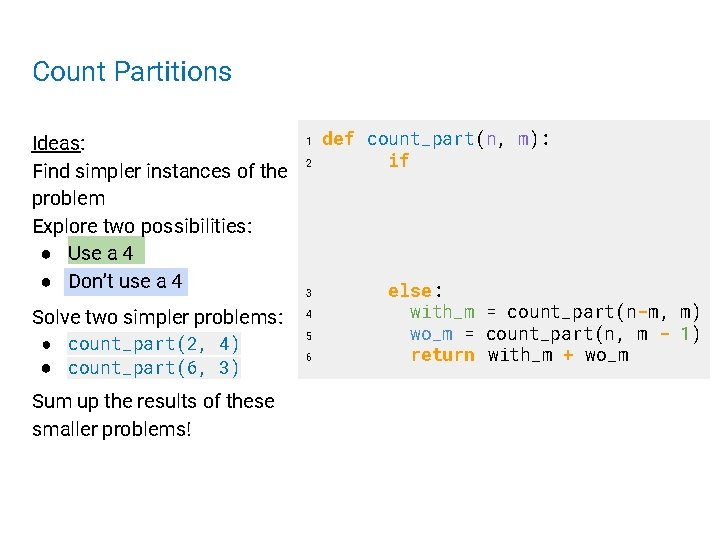Count Partitions Ideas: Find simpler instances of the problem Explore two possibilities: ● Use a 4 ● Don’t use a 4 1 Solve two simpler problems: 4 ● count_part(2, 4) ● count_part(6, 3) Sum up the results of these smaller problems! 2 3 5 6 def count_part(n, m): if else: with_m = count_part(n-m, m) wo_m = count_part(n, m - 1) return with_m + wo_m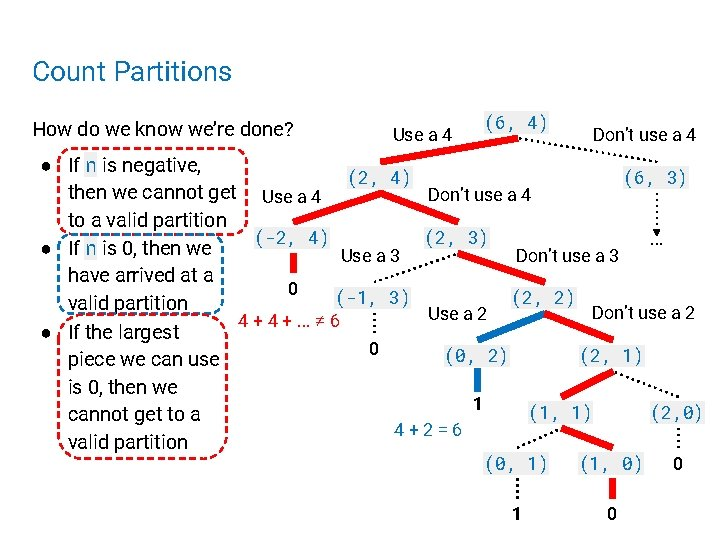Count Partitions How do we know we’re done? Use a 4 (6, 4) Don’t use a 4 ● If n is negative, (2, 4) (6, 3) then we cannot get Use a 4 Don’t use a 4 to a valid partition (-2, 4) (2, 3). . . ● If n is 0, then we Use a 3 Don’t use a 3 have arrived at a 0 (-1, 3) (2, 2) valid partition Don’t use a 2 Use a 2 4 +. . . ≠ 6 ● If the largest 0 (0, 2) (2, 1) piece we can use is 0, then we 1 (1, 1) (2, 0) cannot get to a 4+2=6 valid partition (0, 1) (1, 0) 1 0 0Demo Count Partitions Ideas: Explore two possibilities: ● Use a 4 ● Don’t use a 4 Solve two simpler problems: ● count_part(2, 4) ● count_part(6, 3) def count_part(n, m): 2 if if n == 0: 0: 3 return 1 1 4 elif n < n 0: < 0: 5 return 0 0 elif m == 6 elif m == 0: 0: 0 7 return 0 8 else: 9 with_m = count_part(n-m, = count_part(n m) 10 m, m) wo_m = count_part(n, m - 1) 11 wo_m return = count_part(n, with_m + wo_mo_m m - 1) return with_m + wo_m 1 Sum up the results of these smaller problems! How do we know we’re done? ● If n is 0, then we have arrived at a valid partition ● If n is negative, then we cannot get to a valid partition ● If the largest piece we can use is 0, then we cannot get to a valid partition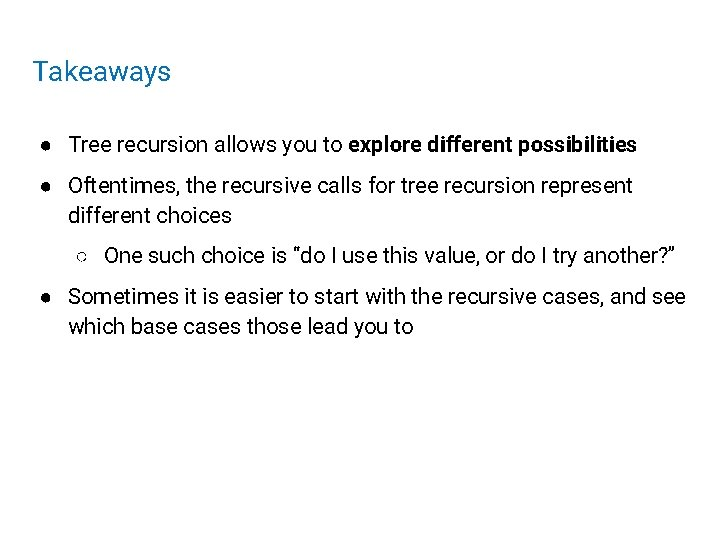Takeaways ● Tree recursion allows you to explore different possibilities ● Oftentimes, the recursive calls for tree recursion represent different choices ○ One such choice is “do I use this value, or do I try another? ” ● Sometimes it is easier to start with the recursive cases, and see which base cases those lead you toIf Time - Speeding Up Recursion (Teaser for the ~Future~)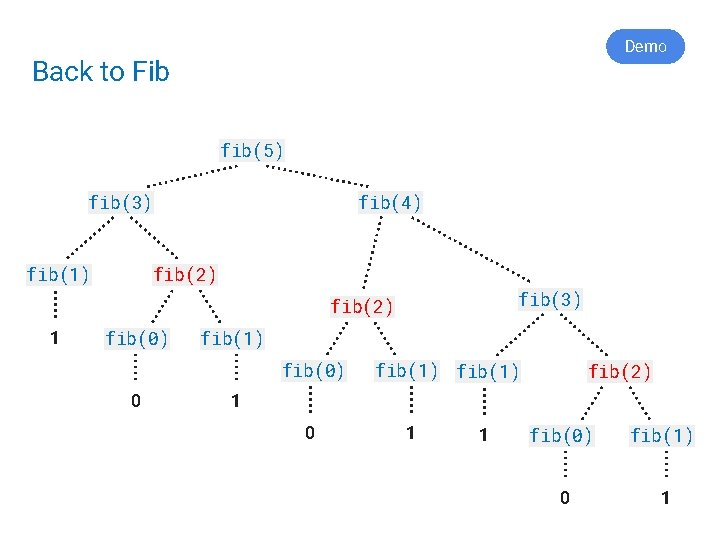Demo Back to Fib fib(5) fib(3) fib(1) fib(4) fib(2) fib(3) fib(2) 1 fib(0) fib(1) fib(0) 0 fib(1) fib(2) 1 0 1 1 fib(0) fib(1) 0 1Basic Idea to Improve: 1 2 3 4 5 6 7 8 9 def better_fib(n): if n == 0: return 0 elif n == 1: return 1 elif already called better_fib(n): return stored value else: store & return better_fib(n - 2) + better_fib(n - 1)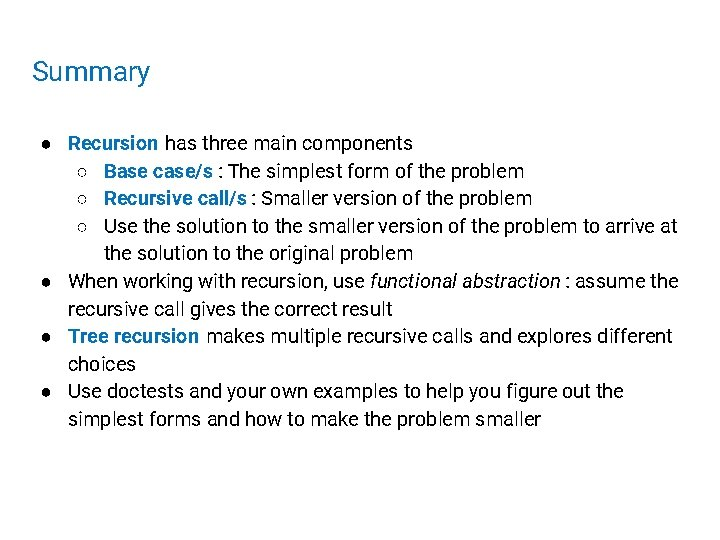Summary ● Recursion has three main components ○ Base case/s : The simplest form of the problem ○ Recursive call/s : Smaller version of the problem ○ Use the solution to the smaller version of the problem to arrive at the solution to the original problem ● When working with recursion, use functional abstraction : assume the recursive call gives the correct result ● Tree recursion makes multiple recursive calls and explores different choices ● Use doctests and your own examples to help you figure out the simplest forms and how to make the problem smaller